## Solving Right Triangles

Triangles are made up of three line segments. They meet to form three angles. The sizes of the angles and the lengths of the sides are related to one another. If you know the size (length) of three out of the six parts of the triangle (at least one side must be included), you can find the sizes of the remaining sides and angles. If the triangle is a right triangle, you can use simple trigonometric ratios to find the missing parts. In a general triangle (acute or obtuse), you need to use other techniques, including the law of cosines and the law of sines. You can also find the area of triangles by using trigonometric ratios.

All triangles are made up of three sides and three angles. If the three angles of the triangle are labeled ∠ A, ∠ B and ∠ C, then the three sides of the triangle should be labeled as a, b, and c. Figure 1  illustrates how lowercase letters are used to name the sides of the triangle that are opposite the angles named with corresponding uppercase letters. If any three of these six measurements are known (other than knowing the measures of the three angles), then you can calculate the values of the other three measurements. The process of finding the missing measurements is known as solving the triangle. If the triangle is a right triangle, then one of the angles is 90°. Therefore, you can solve the right triangle if you are given the measures of two of the three sides or if you are given the measure of one side and one of the other two angles.Figure 1
Drawing for Example 1.

Example 1: Solve the right triangle shown in Figure (b) if ∠ B = 22°

Because the three angles of a triangle must add up to 180°, ∠ A = 90 ∠ B thus ∠ A = 68°.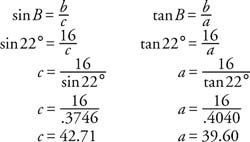The following is an alternate way to solve for sides a and c: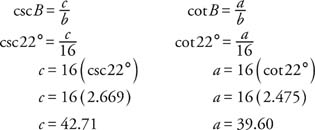This alternate solution may be easier because no division is involved.

Example 2: Solve the right triangle shown in Figure (b) if b = 8 and a = 13.

You can use the Pythagorean theorem to find the missing side, but trigonometric relationships are used instead. The two missing angle measurements will be found first and then the missing side.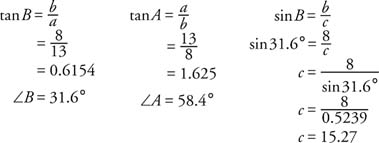In many applications, certain angles are referred to by special names. Two of these special names are angle of elevation and angle of depression. The examples shown in Figure make use of these terms.Figure 2
a) Angle of elevation and b) angle of depression.

Example 3: A large airplane (plane A) flying at 26,000 feet sights a smaller plane (plane B) traveling at an altitude of 24,000 feet. The angle of depression is 40°. What is the line of sight distance ( x) between the two planes?

Figure 3 illustrates the conditions of this problem.Figure 3
Drawing for Example 3.

From Figure 3 , you can find the solution by using the sine of 40 °: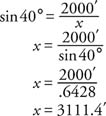Example 4: A ladder must reach the top of a building. The base of the ladder will be 25′ from the base of the building. The angle of elevation from the base of the ladder to the top of the building is 64°. Find the height of the building (h) and the length of the ladder (m).

Figure 4 illustrates the conditions of this problem.Figure 4
Drawing for Example 4.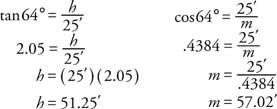Example 5: A woodcutter wants to determine the height of a tall tree. He stands at some distance from the tree and determines that the angle of elevation to the top of the tree is 40°. He moves 30′ closer to the tree, and now the angle of elevation is 50°. If the woodcutter's eyes are 5′ above the ground, how tall is the tree?Figure 5
Drawing for Example 5.

From the small right triangle and from the large right triangle, the following relationships are evident: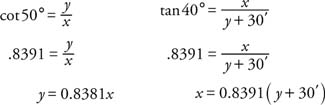Substituting the first equation in the second yields:Note that 5′ must be added to the value of x to get the height of the tree, or 90.06′ tall.

Example 6: Using Figure 6, find the length of sides x and y and the area of the large triangle.Figure 6
Drawing for Example 6.

Because this is an isosceles triangle, and equal sides are opposite equal angles, the values of x and y are the same. If the triangle is divided into two right triangles, the base of each will be 6. Therefore,